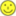# Why is the ohmmeter uneven

## Measure resistances

### Analog ohmmeter

The circuit is simple and the resistance is easy to calculate:

##### Formula 2
R.x = Uv / I

The circuit in Figure 2 has a serious problem. If we measure a very small resistance, e.g. 0.1Ω, then a very high current flows which could destroy the ammeter. A protective resistor is therefore built in.

In the event of a short circuit, Rx= 0Ω, the maximum current through the resistor R1 limited. R.1 is chosen so that the ammeter fully deflects. With a supply voltage of 5V and a maximum current in the ammeter of e.g. 1mA, R would be1= 5V / 1mA = 5kΩ.

The resistance Rx can be calculated with it

##### Formula 3
R.x = (Uv / I) - R1

Formula 3 contains a division and a subtraction.

Simple analog measuring devices are overwhelmed by this, so the problem is left to us humansThe values ​​of the measured resistance are simply entered on the scale of an analog ohmmeter. At 0Ω the ammeter deflects fully, i.e. to the right. If the resistance is very high, only a small current flows and the pointer is on the left.

We only consider the lower scale for Ω

• The values ​​are plotted unevenly
• The values ​​can be estimated more than they can be read.
• But the values ​​1kΩ and 110Ω can be distinguished from each other.